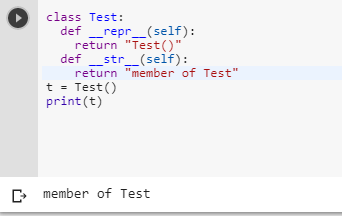4 views
in Python

I am learning the ropes in Python. When I try to print an object of class Foobar using the print()function, I get an output like this:

<__main__.Foobar instance at 0x7ff2a18c>

Is there a way I can set the printing behaviour (or the string representation) of a class and its objects? For instance, when I call to print() on a class object, I would like to print its data members in a certain format. How to achieve this in Python?

If you are familiar with C++ classes, the above can be achieved for the standard ostream by adding a friend ostream& operator << (ostream&, const Foobar&) method for the class.

by (106k points)

For printing objects of a class using print() you can use the __str__ method. The __repr__ method is what happens when you use the repr() function (or when you look at it with the interactive prompt).

If there is no __str__ method is given, Python will print the result of __repr__ instead. If you will define __str__ but not __repr__, Python will use what you see above as the __repr__, but still, use __str__ for printing.

class Test:

def __repr__(self):

return "Test()"

def __str__(self):

return "member of Test"

t = Test()

print(t)